# Creating a new Op: Python implementation¶

So suppose you have looked through the library documentation and you don’t see a function that does what you want.

If you can implement something in terms of an existing Op, you should do that. Odds are your function that uses existing Aesara expressions is short, has no bugs, and potentially profits from optimizations that have already been implemented.

However, if you cannot implement an Op in terms of an existing Op, you have to write a new one.

As an illustration, this tutorial will demonstrate how a simple Python-based Op that performs operations on np.float64s is written.

Note

This is an introductury tutorial and as such it does not cover how to make an Op that returns a view or modifies the values in its inputs. Thus, all Ops created with the instructions described here MUST return newly allocated memory or reuse the memory provided in the parameter output_storage of the Op.perform() method. See Views and inplace operations for an explanation on how to do this.

If your Op returns a view or changes the value of its inputs without doing as prescribed in that page, Aesara will run, but will return correct results for some graphs and wrong results for others.

It is recommended that you run your tests in DebugMode, since it can help verify whether or not your Op behaves correctly in this regard.

## Aesara Graphs refresher¶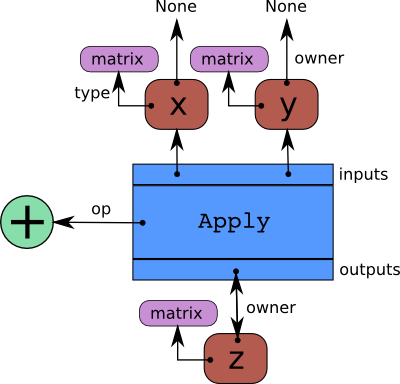Aesara represents symbolic mathematical computations as graphs. Those graphs are bi-partite graphs (graphs with two types of nodes), they are composed of interconnected Apply and Variable nodes. Variable nodes represent data in the graph, either inputs, outputs or intermediary values. As such, inputs and outputs of a graph are lists of Aesara Variable nodes. Apply nodes perform computation on these variables to produce new variables. Each Apply node has a link to an instance of Op which describes the computation to perform. This tutorial details how to write such an Op instance. Please refers to Graph Structures for a more detailed explanation about the graph structure.

## Op’s basic methods¶

An Op is any Python object which inherits from Op. This section provides an overview of the basic methods you typically have to implement to make a new Op. It does not provide extensive coverage of all the possibilities you may encounter or need. For that refer to Ops.

import aesara
from aesara.graph.op import Op

class MyOp(Op):
# Properties attribute
__props__ = ()

#itypes and otypes attributes are
#compulsory if make_node method is not defined.
#They're the type of input and output respectively
itypes = None
otypes = None

#Compulsory if itypes and otypes are not defined
def make_node(self, *inputs):
pass

# Python implementation:
def perform(self, node, inputs_storage, output_storage):
pass

# Other type of implementation
# C implementation: [see aesara web site for other functions]
def c_code(self, node, inputs, outputs, sub):
pass

# Other implementations:
def make_thunk(self, node, storage_map, _, _2, impl=None):
pass

# optional:
check_input = True

def __init__(self, *args):
pass

pass

def R_op(self, inputs, eval_points):
pass

def infer_shape(self, fgraph, node, input_shapes):
pass


An Op has to implement some methods defined in the the interface of Op. More specifically, it is mandatory for an Op to define either the method make_node() or itypes, otypes and one of the implementation methods, either perform(), COp.c_code() or make_thunk().

make_node() method creates an Apply node representing the application of the Op on the inputs provided. This method is responsible for three things:

• it first checks that the input Variables types are compatible with the current Op. If the Op cannot be applied on the provided input types, it must raises an exception (such as TypeError).
• it operates on the Variables found in *inputs in Aesara’s symbolic language to infer the type of the symbolic output Variables. It creates output Variables of a suitable symbolic Type to serve as the outputs of this Op’s application.
• it creates an Apply instance with the input and output Variable, and return the Apply instance.

perform() method defines the Python implementation of an Op. It takes several arguments:

• node is a reference to an Apply node which was previously obtained via the make_node() method. It is typically not used in a simple Op, but it contains symbolic information that could be required by a complex Op.
• inputs is a list of references to data which can be operated on using non-symbolic statements, (i.e., statements in Python, Numpy).
• output_storage is a list of storage cells where the output is to be stored. There is one storage cell for each output of the Op. The data put in output_storage must match the type of the symbolic output. It is forbidden to change the length of the list(s) contained in output_storage. A function Mode may allow output_storage elements to persist between evaluations, or it may reset output_storage cells to hold a value of None. It can also pre-allocate some memory for the Op to use. This feature can allow perform to reuse memory between calls, for example. If there is something preallocated in the output_storage, it will be of the good dtype, but can have the wrong shape and have any stride pattern.

perform() method must be determined by the inputs. That is to say, when applied to identical inputs the method must return the same outputs.

An Ops implementation can be defined in other ways, as well. For instance, it is possible to define a C-implementation via COp.c_code(). Please refers to tutorial Extending Aesara with a C Op for a description of COp.c_code() and other related c_** methods. Note that an Op can provide both Python and C implementations.

make_thunk() method is another alternative to perform(). It returns a thunk. A thunk is defined as a zero-arguments function which encapsulates the computation to be performed by an Op on the arguments of its corresponding node. It takes several parameters:

• node is the Apply instance for which a thunk is requested,
• storage_map is a dict of lists which maps variables to a one-element lists holding the variable’s current value. The one-element list acts as pointer to the value and allows sharing that “pointer” with other nodes and instances.
• compute_map is also a dict of lists. It maps variables to one-element lists holding booleans. If the value is 0 then the variable has not been computed and the value should not be considered valid. If the value is 1 the variable has been computed and the value is valid. If the value is 2 the variable has been garbage-collected and is no longer valid, but shouldn’t be required anymore for this call. The returned function must ensure that it sets the computed variables as computed in the compute_map.
• impl allow to select between multiple implementation. It should have a default value of None.

make_thunk() is useful if you want to generate code and compile it yourself.

If make_thunk() is defined by an Op, it will be used by Aesara to obtain the Op’s implementation. perform() and COp.c_code() will be ignored.

If make_node() is not defined, the itypes and otypes are used by the Op’s make_node() method to implement the functionality of make_node() method mentioned above.

## Op’s auxiliary methods¶

There are other methods that can be optionally defined by the Op:

The __str__() method provides a meaningful string representation of your Op.

__eq__() and __hash__() define respectivelly equality between two Ops and the hash of an Op instance. They will be used by the optimization phase to merge nodes that are doing equivalent computations (same inputs, same operation). Two Ops that are equal according __eq__() should return the same output when they are applied on the same inputs.

The __props__ attribute lists the properties that influence how the computation is performed (usually these are set in __init__()). It must be a tuple. If you don’t have any properties, then you should set this attribute to the empty tuple ().

__props__ enables the automatic generation of appropriate __eq__() and __hash__(). Given the method __eq__(), automatically generated from __props__, two Ops will be equal if they have the same values for all the properties listed in __props__. Given to the method __hash__() automatically generated from __props__, two Ops will be have the same hash if they have the same values for all the properties listed in __props__. __props__ will also generate a suitable __str__() for your Op. This requires development version after September 1st, 2014 or version 0.7.

The infer_shape() method allows an Op to infer the shape of its output variables without actually computing them. It takes as input fgraph, a FunctionGraph; node, a reference to the Op’s Apply node; and a list of Variabless (e.g. i0_shape, i1_shape, …) which are the dimensions of the Op input Variables. infer_shape() returns a list where each element is a tuple representing the shape of one output. This could be helpful if one only needs the shape of the output instead of the actual outputs, which can be useful, for instance, for optimization procedures.

The grad() method is required if you want to differentiate some cost whose expression includes your Op. The gradient may be specified symbolically in this method. It takes two arguments inputs and output_gradients, which are both lists of Variables, and those must be operated on using Aesara’s symbolic language. The grad() method must return a list containing one Variable for each input. Each returned Variable represents the gradient with respect to that input computed based on the symbolic gradients with respect to each output. If the output is not differentiable with respect to an input then this method should be defined to return a variable of type NullType for that input. Likewise, if you have not implemented the grad computation for some input, you may return a variable of type NullType for that input. Please refer to grad() for a more detailed view.

The R_op() method is needed if you want aesara.gradient.Rop to work with your Op. This function implements the application of the R-operator on the function represented by your Op. Let assume that function is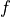, with input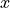, applying the R-operator means computing the Jacobian ofand right-multiplying it by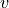, the evaluation point, namely: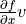.

The optional boolean check_input attribute is used to specify if you want the types used in your COp to check their inputs in their COp.c_code(). It can be used to speed up compilation, reduce overhead (particularly for scalars) and reduce the number of generated C files.

## Example: Op definition¶

import aesara
from aesara.graph.op import Op
from aesara.graph.basic import Apply

class DoubleOp1(Op):
__props__ = ()

def make_node(self, x):
x = aesara.tensor.as_tensor_variable(x)
# Note: using x_.type() is dangerous, as it copies x's broadcasting
# behaviour
return Apply(self, [x], [x.type()])

def perform(self, node, inputs, output_storage):
x = inputs
z = output_storage
z = x * 2

def infer_shape(self, fgraph, node, i0_shapes):
return i0_shapes

def R_op(self, inputs, eval_points):
# R_op can receive None as eval_points.
# That mean there is no diferientiable path through that input
# If this imply that you cannot compute some outputs,
# return None for those.
if eval_points is None:
return eval_points

doubleOp1 = DoubleOp1()

#Using itypes and otypes

class DoubleOp2(Op):
__props__ = ()

itypes = [aesara.tensor.dmatrix]
otypes = [aesara.tensor.dmatrix]

def perform(self, node, inputs, output_storage):
x = inputs
z = output_storage
z = x * 2

def infer_shape(self, fgraph, node, i0_shapes):
return i0_shapes

def R_op(self, inputs, eval_points):
# R_op can receive None as eval_points.
# That mean there is no diferientiable path through that input
# If this imply that you cannot compute some outputs,
# return None for those.
if eval_points is None:
return eval_points

doubleOp2 = DoubleOp2()


At a high level, the code fragment declares a class (e.g., DoubleOp1) and then creates one instance of it (e.g., doubleOp1).

We often gloss over this distinction, but will be precise here: doubleOp1 (the instance) is an Op, not DoubleOp1 (the class which is a subclass of Op). You can call doubleOp1(tensor.vector()) on a Variable to build an expression, and in the expression there will be a .op attribute that refers to doubleOp1.

The make_node method creates a node to be included in the expression graph. It runs when we apply our Op (doubleOp1) to the Variable (x), as in doubleOp1(tensor.vector()). When an Op has multiple inputs, their order in the inputs argument to Apply is important: Aesara will call make_node(*inputs) to copy the graph, so it is important not to change the semantics of the expression by changing the argument order.

All the inputs and outputs arguments to Apply must be Variables. A common and easy way to ensure inputs are variables is to run them through as_tensor_variable. This function leaves TensorType variables alone, raises an error for non-TensorType variables, and copies any numpy.ndarray into the storage for a TensorType Constant. The make_node() method dictates the appropriate Type for all output variables.

The perform() method implements the Op’s mathematical logic in Python. The inputs (here x) are passed by value, but a single output is returned indirectly as the first element of single-element lists. If doubleOp1 had a second output, it would be stored in output_storage.

In some execution modes, the output storage might contain the return value of a previous call. That old value can be reused to avoid memory re-allocation, but it must not influence the semantics of the Op output.

You can try the new Op as follows:

import numpy as np
import aesara

x = aesara.tensor.matrix()
f = aesara.function([x], DoubleOp1()(x))
inp = np.random.random_sample((5, 4))
out = f(inp)
assert np.allclose(inp * 2, out)
print(inp)
print(out)

[[ 0.08257206  0.34308357  0.5288043   0.06582951]
[ 0.65977826  0.10040307  0.5402353   0.55472296]
[ 0.82358552  0.29502171  0.97387481  0.0080757 ]
[ 0.77327215  0.65401857  0.76562992  0.94145702]
[ 0.8452076   0.30500101  0.88430501  0.95818655]]
[[ 0.16514411  0.68616713  1.0576086   0.13165902]
[ 1.31955651  0.20080613  1.08047061  1.10944593]
[ 1.64717104  0.59004341  1.94774962  0.0161514 ]
[ 1.5465443   1.30803715  1.53125983  1.88291403]
[ 1.6904152   0.61000201  1.76861002  1.9163731 ]]

import numpy as np
import aesara

x = aesara.tensor.matrix()
f = aesara.function([x], DoubleOp2()(x))
inp = np.random.random_sample((5, 4))
out = f(inp)
assert np.allclose(inp * 2, out)
print(inp)
print(out)

[[ 0.02443785  0.67833979  0.91954769  0.95444365]
[ 0.60853382  0.7770539   0.78163219  0.92838837]
[ 0.04427765  0.37895602  0.23155797  0.4934699 ]
[ 0.20551517  0.7419955   0.34500905  0.49347629]
[ 0.24082769  0.49321452  0.24566545  0.15351132]]
[[ 0.04887571  1.35667957  1.83909538  1.90888731]
[ 1.21706764  1.55410779  1.56326439  1.85677674]
[ 0.08855531  0.75791203  0.46311594  0.9869398 ]
[ 0.41103034  1.48399101  0.69001811  0.98695258]
[ 0.48165539  0.98642904  0.4913309   0.30702264]]


## Example: __props__ definition¶

We can modify the previous piece of code in order to demonstrate the usage of the __props__ attribute.

We create an Op that takes a variable x and returns a*x+b. We want to say that two such Ops are equal when their values of a and b are equal.

import aesara
from aesara.graph.op import Op
from aesara.graph.basic import Apply

class AXPBOp(Op):
"""
This creates an Op that takes x to a*x+b.
"""
__props__ = ("a", "b")

def __init__(self, a, b):
self.a = a
self.b = b
super().__init__()

def make_node(self, x):
x = aesara.tensor.as_tensor_variable(x)
return Apply(self, [x], [x.type()])

def perform(self, node, inputs, output_storage):
x = inputs
z = output_storage
z = self.a * x + self.b

def infer_shape(self, fgraph, node, i0_shapes):
return i0_shapes



The use of __props__ saves the user the trouble of implementing __eq__() and __hash__() manually. It also generates a default __str__() method that prints the attribute names and their values.

We can test this by running the following segment:

mult4plus5op = AXPBOp(4, 5)
another_mult4plus5op = AXPBOp(4, 5)
mult2plus3op = AXPBOp(2, 3)

assert mult4plus5op == another_mult4plus5op
assert mult4plus5op != mult2plus3op

x = aesara.tensor.matrix()
f = aesara.function([x], mult4plus5op(x))
g = aesara.function([x], mult2plus3op(x))

inp = np.random.random_sample((5, 4)).astype(np.float32)
assert np.allclose(4 * inp + 5, f(inp))
assert np.allclose(2 * inp + 3, g(inp))


## How To Test it¶

Aesara has some functionalities to simplify testing. These help test the infer_shape(), grad() and R_op() methods. Put the following code in a file and execute it with the pytest program.

### Basic Tests¶

Basic tests are done by you just by using the Op and checking that it returns the right answer. If you detect an error, you must raise an exception. You can use the assert keyword to automatically raise an AssertionError.

import numpy as np
import aesara
from tests import unittest_tools as utt

class TestDouble(utt.InferShapeTester):
def setup_method(self):
super().setup_method()
self.op_class = DoubleOp
self.op = DoubleOp()

def test_basic(self):
rng = np.random.default_rng(utt.fetch_seed())

x = aesara.tensor.matrix()
f = aesara.function([x], self.op(x))

inp = np.asarray(rng.random((5, 4)), dtype=aesara.config.floatX)
out = f(inp)
# Compare the result computed to the expected value.
utt.assert_allclose(inp * 2, out)


We call utt.assert_allclose(expected_value, value) to compare NumPy ndarray.This raise an error message with more information. Also, the default tolerance can be changed with the Aesara flags config.tensor__cmp_sloppy that take values in 0, 1 and 2. The default value do the most strict comparison, 1 and 2 make less strict comparison.

### Testing the infer_shape¶

When a class inherits from the InferShapeTester class, it gets the InferShapeTester._compile_and_check() method that tests the Op.infer_shape() method. It tests that the Op gets optimized out of the graph if only the shape of the output is needed and not the output itself. Additionally, it checks that the optimized graph computes the correct shape, by comparing it to the actual shape of the computed output.

InferShapeTester._compile_and_check() compiles an Aesara function. It takes as parameters the lists of input and output Aesara variables, as would be provided to aesara.function(), and a list of real values to pass to the compiled function. It also takes the Op class as a parameter in order to verify that no instance of it appears in the shape-optimized graph.

If there is an error, the function raises an exception. If you want to see it fail, you can implement an incorrect Op.infer_shape().

When testing with input values with shapes that take the same value over different dimensions (for instance, a square matrix, or a tensor3 with shape (n, n, n), or (m, n, m)), it is not possible to detect if the output shape was computed correctly, or if some shapes with the same value have been mixed up. For instance, if the infer_shape uses the width of a matrix instead of its height, then testing with only square matrices will not detect the problem. This is why the InferShapeTester._compile_and_check() method prints a warning in such a case. If your Op works only with such matrices, you can disable the warning with the warn=False parameter.

from aesara.configdefaults import config
from tests import unittest_tools as utt

class TestDouble(utt.InferShapeTester):

# [...] as previous tests.

def test_infer_shape(self):
rng = np.random.default_rng(utt.fetch_seed())
x = aesara.tensor.matrix()
self._compile_and_check(
[x],  # aesara.function inputs
[self.op(x)],  # aesara.function outputs
# Always use not square matrix!
# inputs data
[np.asarray(rng.random((5, 4)), dtype=config.floatX)],
# Op that should be removed from the graph.
self.op_class,
)


The function verify_grad verifies the gradient of an Op or Aesara graph. It compares the analytic (symbolically computed) gradient and the numeric gradient (computed through the Finite Difference Method).

If there is an error, the function raises an exception. If you want to see it fail, you can implement an incorrect gradient (for instance, by removing the multiplication by 2).

def test_grad(self):
rng = np.random.default_rng(utt.fetch_seed())
self.op,
[rng.random((5, 7, 2))]
)


### Testing the Rop¶

The class RopLop_checker defines the functions RopLop_checker.check_mat_rop_lop(), RopLop_checker.check_rop_lop() and RopLop_checker.check_nondiff_rop(). These allow to test the implementation of the Rop() method of a particular Op.

For instance, to verify the Rop() method of the DoubleOp, you can use this:

import numpy
import tests
from tests.test_rop import RopLop_checker
class TestDoubleRop(RopLop_checker):
def setUp(self):
super(TestDoubleRop, self).setUp()
def test_double_rop(self):
self.check_rop_lop(DoubleRop()(self.x), self.in_shape)


To perform your tests, simply run pytest.

#### In-file¶

One may also add a block of code similar to the following at the end of the file containing a specific test of interest and run the file. In this example, the test TestDoubleRop in the class test_double_op would be performed.

if __name__ == '__main__':
t = TestDoubleRop("test_double_rop")
t.setUp()
t.test_double_rop()


We recommend that when we execute a file, we run all tests in that file. This can be done by adding this at the end of your test files:

if __name__ == '__main__':
unittest.main()


#### Exercise¶

Run the code of the DoubleOp example above.

Modify and execute to compute: x * y.

Modify and execute the example to return two outputs: x + y and jx - yj.

You can omit the Rop() functions. Try to implement the testing apparatus described above.

(Notice that Aesara’s current elemwise fusion optimization is only applicable to computations involving a single output. Hence, to gain efficiency over the basic solution that is asked here, the two operations would have to be jointly optimized explicitly in the code.)

#### Random numbers in tests¶

Making tests errors more reproducible is a good practice. To make your tests more reproducible, you need a way to get the same random numbers. You can do this by seeding NumPy’s random number generator.

For convenience, the classes InferShapeTester and RopLop_checker already do this for you. If you implement your own setUp() method, don’t forget to call the parent setUp() method.

Solution

## as_op()¶

as_op() is a Python decorator that converts a Python function into a basic Aesara Op that will call the supplied function during execution.

This isn’t the recommended way to build an Op, but allows for a quick implementation.

It takes an optional infer_shape() parameter that must have this signature:

  def infer_shape(fgraph, node, input_shapes):
# ...
return output_shapes

- :obj:input_shapes and :obj:output_shapes are lists of tuples that
represent the shape of the corresponding inputs/outputs, and :obj:fgraph
is a :class:FunctionGraph.


Warning

Not providing a infer_shape prevents shape-related optimizations from working with this Op. For example your_op(inputs, ...).shape will need the Op to be executed just to get the shape.

Note

As no grad is defined, this means you won’t be able to differentiate paths that include this Op.

Note

It converts the Python function to a callable object that takes as inputs Aesara variables that were declared.

Note

The python function wrapped by the as_op() decorator needs to return a new data allocation, no views or in place modification of the input.

### as_op() Example¶

import aesara
import aesara.tensor as at
import numpy as np
from aesara import function
from aesara.compile.ops import as_op

def infer_shape_numpy_dot(fgraph, node, input_shapes):
ashp, bshp = input_shapes
return [ashp[:-1] + bshp[-1:]]

@as_op(itypes=[at.matrix, at.matrix],
otypes=[at.matrix], infer_shape=infer_shape_numpy_dot)
def numpy_dot(a, b):
return np.dot(a, b)


You can try it as follows:

x = at.matrix()
y = at.matrix()
f = function([x, y], numpy_dot(x, y))
inp1 = np.random.random_sample((5, 4))
inp2 = np.random.random_sample((4, 7))
out = f(inp1, inp2)


## Documentation and Coding Style¶

Please always respect the Requirements for Quality Contributions or your contribution will not be accepted.

## NanGuardMode and AllocEmpty¶

NanGuardMode help users find where in the graph NaN appear. But sometimes, we want some variables to not be checked. For example, in the old GPU back-end, we used a float32 CudaNdarray to store the MRG random number generator state (they are integers). So if NanGuardMode checked it, it would generate a false positive. Another case is related to AllocEmpty or some computations on it (like done by Scan).

You can tell NanGuardMode to do not check a variable with: variable.tag.nan_guard_mode_check. Also, this tag automatically follow that variable during optimization. This mean if you tag a variable that get replaced by an inplace version, it will keep that tag.

## Final Note¶

A more extensive discussion of this section’s content may be found in the advanced tutorial Extending Aesara.

The section Other Ops includes more instructions for the following specific cases: